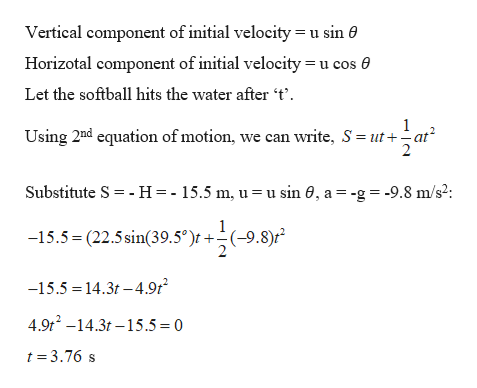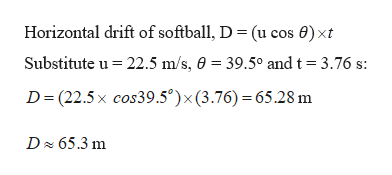A physics student stands on a cliff overlooking a lake and decides to throw a softball to her friends in the water below. She throws the softball with a velocity of 22.5 m/s22.5 m/s at an angle of 39.5∘39.5∘ above the horizontal. When the softball leaves her hand, it is 15.5 m15.5 m above the water. How far does the softball travel horizontally before it hits the water? Neglect any effects of air resistance when calculating the answer.

Question

A physics student stands on a cliff overlooking a lake and decides to throw a softball to her friends in the water below. She throws the softball with a velocity of 22.5 m/s22.5 m/s at an angle of 39.5∘39.5∘ above the horizontal. When the softball leaves her hand, it is 15.5 m15.5 m above the water. How far does the softball travel horizontally before it hits the water? Neglect any effects of air resistance when calculating the answer.

Step 1

Given:

Velocity of ball thrown, u = 22.5 m/s

Initial height of the ball above the water, H = 15.5 m

Angle of projection, θ = 39.5o

Step 2

Calculating the time taken by the ball to reach to the water:help_outlineImage TranscriptioncloseVertical component of initial velocity u sin 0 Horizotal component of initial velocity= u cos e Let the softball hits the water after 't' Using 2nd equation of motion, we can write, S utat - H=- 15.5 m, u = u sin 0, a -g 9.8 m/s?: Substitute S -15.5 (22.5 sin(39.5')r +-(-9.8)r -15.5 14.3t -4.9r 4.9t2 -14.3t -15.5 =0 t 3.76 s fullscreen
Step 3

Calculating the horizontal distance travelled b...help_outlineImage TranscriptioncloseHorizontal drift of softball, D = (u cos 0) xt Substitute u 3.76 s 22.5 m/s, 0 39.5° and t D (22.5x cos39.5°) x (3.76) 65.28 m D 65.3 m fullscreen

Want to see the full answer?

See Solution

Want to see this answer and more?

Our solutions are written by experts, many with advanced degrees, and available 24/7

See Solution
Tagged in

Kinematics